# NCERT Solutions for Class 10 Math Chapter 1 - Real Numbers

Aasoka offers NCERT Solutions for Class 10th Math for free. The experts prepare these solutions in a simple and lucid language so that they can easily be understood by students. They are a huge help while students are gearing up for their board exam preparation. The NCERT Solutions are designed as per the latest exam pattern and syllabus. With the help of these solutions, students of Class 10th can learn to frame the answers as per the given marking scheme of the board exams.

“Real Numbers” chapter of Math Class 10th will make students learn and understand irrational numbers and real numbers. At the start of the chapter, students will get to know about Euclid’s Division Lemma which states that for any 2 positive integers, suppose ‘a’ and ‘b’, the condition ‘a = bq + r’, where 0≤ r < b will always hold true. A lemma means a statement that has already been proved. Euclid’s Division Lemma has been devised by a Greek mathematician, Euclid. After this topic, the Fundamental Theorem of Arithmetic is being discussed which helps in finding the HCF and LCM of two positive integers. Then, the decimal expansion of rational numbers, and rational and irrational numbers are described with the help of the theorem.

##### Question 1:

Use Euclid’s division algorithm to find the HCF of:

1. 135 and 225
2. 196 and 38220
3. 867 and 255
1. By division Algorithm

Step 1: Since 225 > 135, we apply the division lemma to 225 and 135, we get 225 = 135 x 1 + 90

Step 2: Since the remainder 90 $\ne$ 0, we apply the division lemma to 135 and 90, we get 135 = 90 x 1 + 45

Step 3: Since the remainder 45 $\ne$ 0, we apply the division lemma to 90 and 45, we get 90 = 45 x 2 + 0

Since the remainder has now become zero, so we stop the procedure.

∵ Divisor in the step 3 is 45.

∴ HCF of 90 and 45 is 45.

Hence, HCF of 135 and 225 is 45.

2. Hence, HCF of 135 and 225 is 45.

Since, 38220 > 196, we apply the division lemma to 196 and 38220, we get 38220 = 196 x 195 + 0

Since the remainder has now become zero, so we stop the procedure.

∵ Divisor in the step 1 is 196

∴ HCF of 38220 and 196 is 196.

Hence, HCF of 38220 and 196 is 196.

3. To find HCF of 867 and 255

Step 1: Since 867 > 255, we apply the division lemma to 867 and 255, we get 867 = 255 x 3 + 102

Step 2: Since remainder 102 $\ne$ 0, we apply the division lemma to 255 and 102, we get 255 = 102 x 2 + 51

Step 3: Since remainder 51 $\ne$ 0, we apply thedivision lemma to 51 and 102, by taking 102 as dividend, we get 102 = 51 x 2 + 0

Since the remainder has now become zero, so we stop the procedure.

∵ Divisor in step 3 is 51

∴ HCF of 102 and 51 is 51.

∴ HCF of 102 and 51 is 51.

##### Question 2:

Show that any positive odd integer is of the form 6q + 1 or 6q + 3 or 6q + 5, where q is some integer.

Let a be any positive odd integer, we apply the division algorithm with a and b = 6.

Since 0 $\le$ r $<$ 6, the possible remainders are 0, 1, 2, 3, 4 and 5.

i.e., a can be 6q or 6q + 1, or 6q + 2, or 6q + 3, or 6q + 4, or 6q + 5 where q is quotient.

However, since a is odd

∴ a cannot be equal to 6q, 6q + 2, 6q + 4

∴ a cannot be equal to 6q, 6q + 2, 6q + 4

Therefore, any odd integer is of the form 6q + 1
or 6q + 3 or 6q + 5.

##### Question 3:

An army contingent of 616 members is to march behind an army band of 32 members in a parade. The two groups are to march in the same number of columns. What is the maximum number of columns in which they can march?

Total number of members in army = 616 and 32 (A band of two groups)

Since two groups are to march in same number of columns and we are to find out the maximum number of columns.

∴ Maximum number of columns = HCF of 616 and 32

Step 1: Since 616 > 32, we apply the division lemma to 616 and 32, to get 616 = 32 x 19 + 8

Step 2: Since the remainder 8 ≠ 0, we apply the division lemma to 32 and 8, to get 32 = 8 x 4 + 0
Since the remainder has now become zero, so we stop the procedure.

∵ Divisor in the last step is 8

∴ HCF of 616 and 32 is 8

Hence, maximum number of columns in which they can march is 8.

##### Question 4:

Use Euclid’s division lemma to show that the square of any positive integer is either of the form 3m or 3m + 1 for some integer m.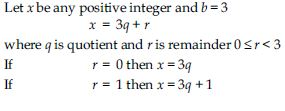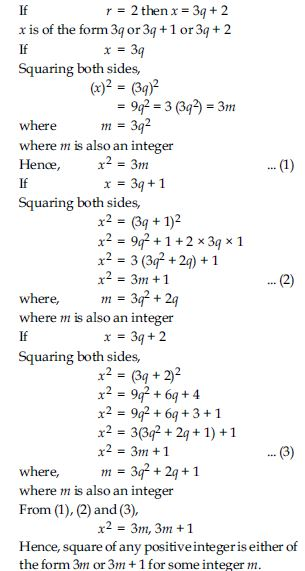##### Question 5:

Use Euclid’s division lemma to show that the cube of any positive integer is of the form 9m, 9m + 1 or 9m + 8.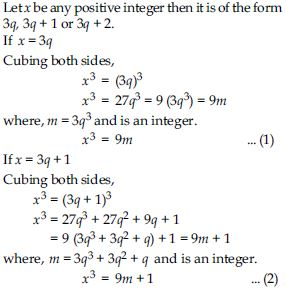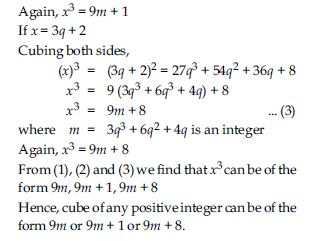##### Question 6:

Express each number as a product of its prime factors:

1. 140
2. 156
3. 3825
4. 5005
5. 7429
1. Prime factorisation of 140 = (2)2 x (35)

Prime factorisation of 140 = (2)2 x (35)

2. Prime factorisation of 156 = (2)2 x (39)

= (2)2 x (3) x (13)

3. Prime factorisation of 3825 = (3)2 x (425)

= (3)2 x (5) x (85)

= (3)2 x (5)2 x (17)

4. Prime factorisation of 5005 = (5) x (1001)

= (5) x (7) x (143)

= (5) x (7) x (11) x (13)

5. = (5) x (7) x (11) x (13)

= (17) x (19) x (23).

##### Question 7:

Find the LCM and HCF of the following pairs of integers and verify that LCM x HCF = Product of the two numbers.

1. 26 and 91
2. 510 and 92
3. 336 and 54
1. Given numbers are 26 and 91

Prime factorisation of 26 and 91 are
26 = (2) x (13)
and 91 = (7) x (13)
HCF (26, 91) = Product of least powers of common factors
∴ HCF (26, 91) = 13
and LCM (26, 91) = Product of highest powers of all the factors
= (2) x (7) x (13) = 182
Verification:
LCM (26, 91) x HCF (26, 91) = (13) x (182)
= (13) x (2) x (91) = (26) x (91)
= Product of given numbers

2. Given numbers are 510 and 92

Prime factorisation of 510 and 92 are
510 = (2) x (255) = (2) x (3) x (85)
= (2) x (3) x (5) x (17)
and 92 = (2) x (46) = (2)2 x (23)
HCF (510, 92) = Product of least powers of common factors = 2
LCM (510, 92) = Product of highest powers of all the factors
= (2)2 x (3) x (5) x (17) x (23) = 23460
Verification:
LCM (510, 92) x HCF (510, 92)
= (2) x (23460)
= (2) x (2)2 x (3) x (5) x (17) x (23)
= (2) x (3) x (5) x (17) x (2)2 x (23)
= 510 x 92
= Product of given numbers

3. Given numbers are 336 and 54

Prime factorisation of 336 and 54 are
336 = (2) x (168) = (2) x (2) x (84)
= (2) x (2) x (2) x (42)
= (2) x (2) x (2) x (2) x (21)
= (2)4 x (3) x (7)
and 54 = (2) x (27) = (2) x (3) x (9)
= (2) x (3) x (3) x (3) = (2) x (3)3
HCF (336, 54) = Product of least powers of common factors
= (2) x (3) = 6
LCM (336, 54) = Product of highest powers of all the factors
= (2)4 x (3)3 x (7) = 3024
Verification:
LCM (336, 54) x HCF (336, 54)
= 6 x 3024 = (2) x (3) x (2)4 x (3)3 x (7)
= (2)4 x (3) x (7) x (2) x (3)3 = 336 x 54
= Product of given numbers.

##### Question 8:

Find the LCM and HCF of the following
integers by applying the prime factorisation method.

1. 12, 15 and 21
2. 17, 23 and 29
3. 8, 9 and 25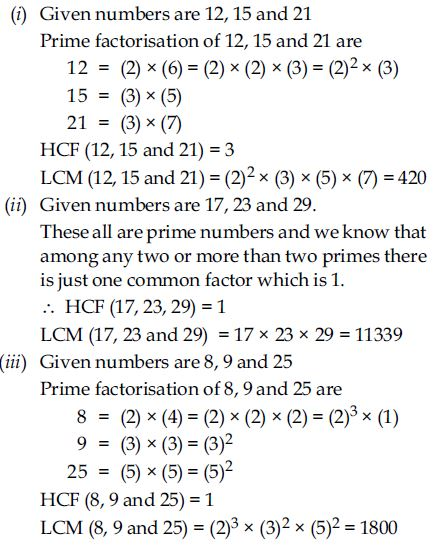##### Question 9:

Given that HCF (306, 657) = 9, find LCM (306, 657).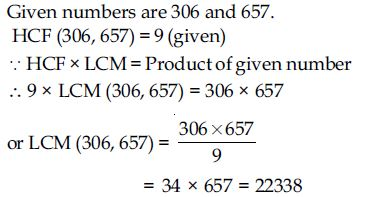##### Question 10:

Check whether 6n can end with the digit 0 for any natural number n.

Let us suppose that 6n ends with the digit 0 for some n $\in$ N.

∴ 6n is divisible by 5.
But, prime factors of 6 are 2 and 3
∴ Prime factors of (6)n are (2 x 3)n
$⇒$ It is clear that in prime factorisation of 6n there is no place for 5.
∵ By Fundamental Theorem of Arithmetic,
Every composite number can be expressed as a product of primes and this factorisation is unique, apart from the order in which the prime factors occur.
∴ Our supposition is wrong.
Hence, there exists no natural number n for which 6n ends with the digit zero.

##### Question 11:

Explain why 7 x 11 x 13 + 13 and 7 x 6 x 5 x 4 x 3 x 2 x 1 + 5 are composite numbers.

Consider, 7 x 11 x 13 + 13 = 13 [7 x 11 + 1]
which is not a prime number because it has a factor 13. So, it is a composite number.
Also, 7 x 6 x 5 x 4 x 3 x 2 x 1 + 5
= 5 [7 x 6 x 4 x 3 x 2 x 1 + 1], which is not a prime number because it has a factor 5. So it is a composite number.

##### Question 12:

There is a circular path around a sports field. Sonia takes 18 minutes to drive one round of the field, while Ravi takes 12 minutes for the same. Suppose they both start at the same point and at the same time, and go in the same direction. After how many minutes will they meet again at the starting point?

Time taken by Sonia to drive one round of the field = 18 minutes

Time taken by Ravi to drive one round of same field = 12 minutes

They meet again at the starting point = LCM (18, 12)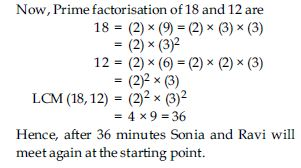##### Question 13: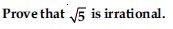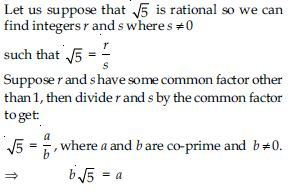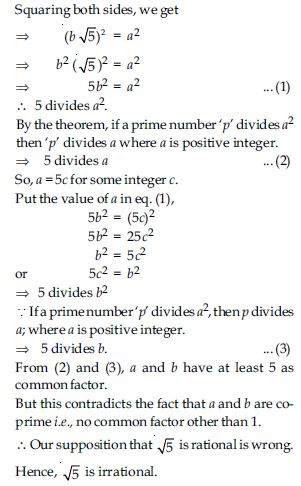##### Question 14: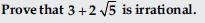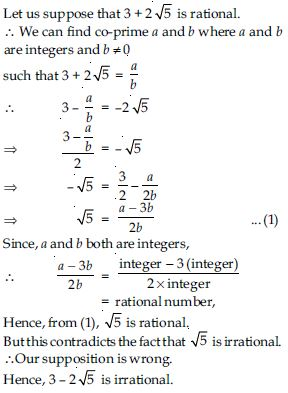##### Question 15: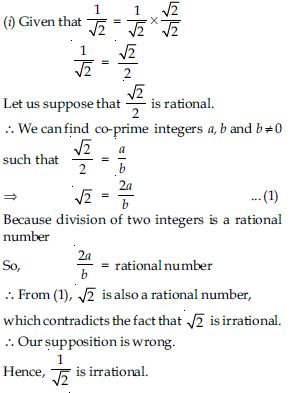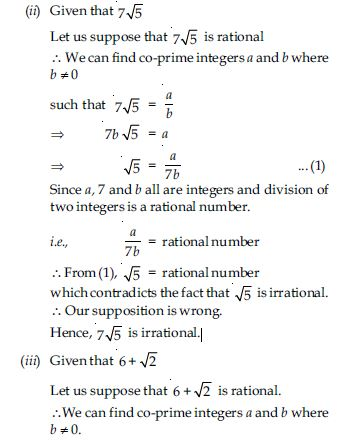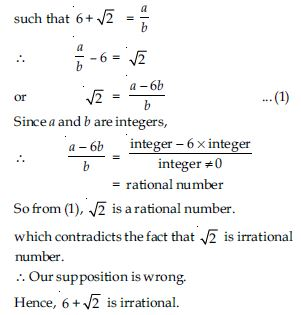##### Question 16: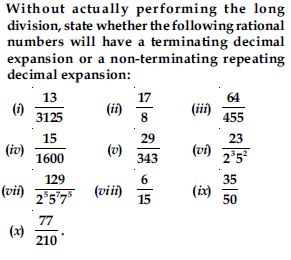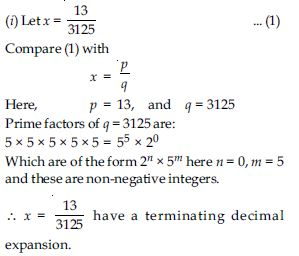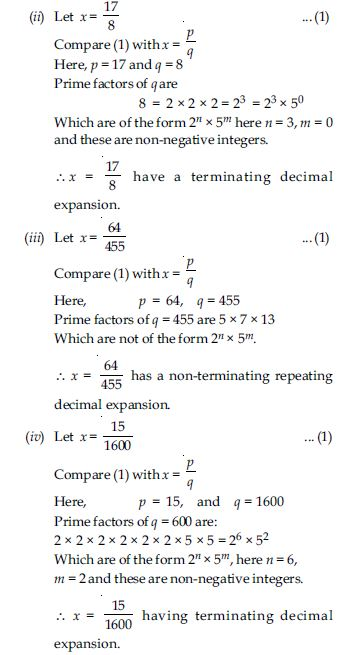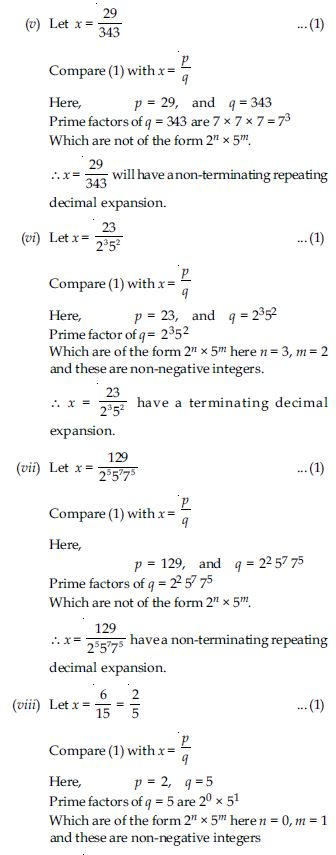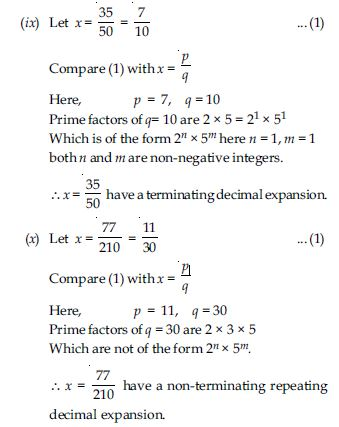##### Question 17:

Write down the decimal expansions of those rational numbers which have terminating decimal expansions.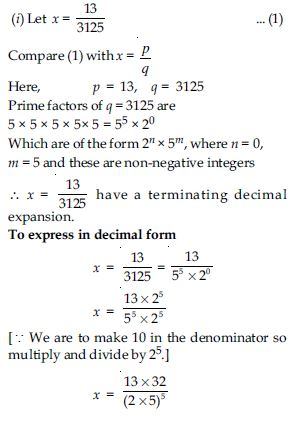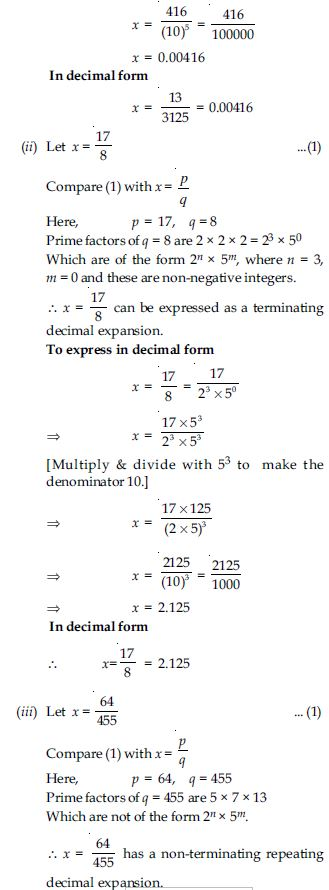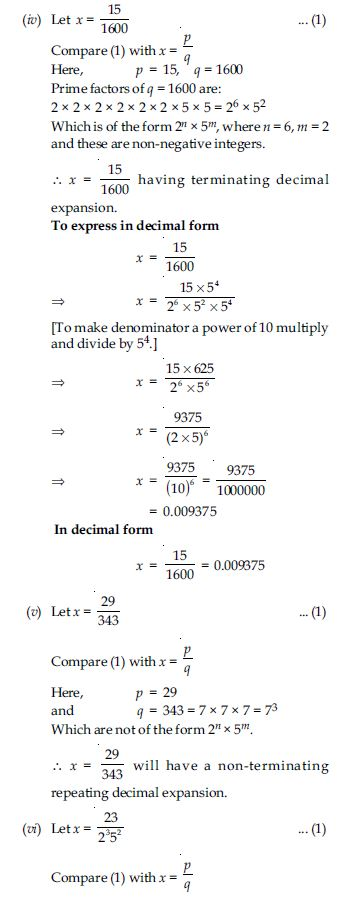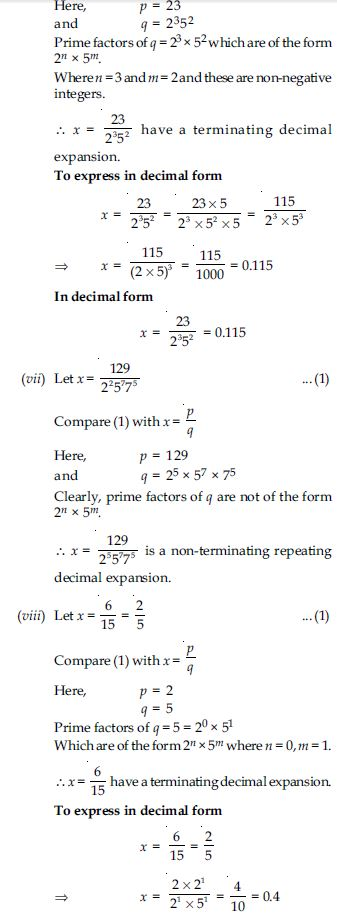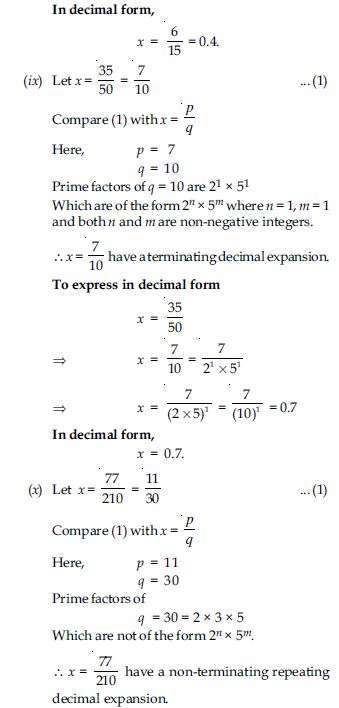##### Question 18: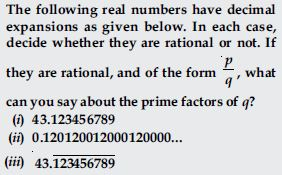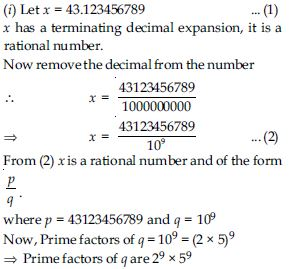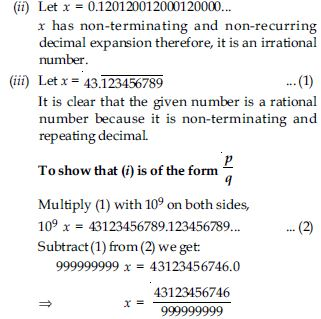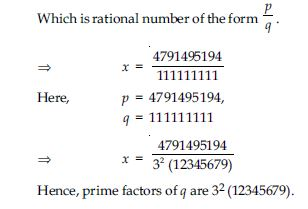##### Question 20:

For some integer m, every even integer is of the form:

1. m
2. m + 1
3. 2m
4. 2m + 1

(c) 2m

##### Question 21:

For some integer q, every odd integer is of the form:

1. q
2. q + 1
3. 2q
4. 2q + 1

(d) 2q + 1

##### Question 22:

n2 - 1 is divisible by 8, if n is:

1. an integer
2. a natural number
3. an odd integer
4. an even integer

(c) an odd integer

##### Question 23:

If the HCF of 65 and 117 is expressible in the form 65 m - 117, then the value of m is:

1. 4
2. 2
3. 1
4. 3

(b) 2

##### Question 24:

The largest number which divides 70 and 125, leaving remainders 5 and 8, respectively is:

1. 13
2. 65
3. 875
4. 1750

(a) 13

##### Question 25:

If two positive integers a and b are written as
a = x3 y2 and b = xy3; x, y are prime numbers, then HCF (a, b) is:

1. xy
2. xy2
3. x3y3
4. x2y2

(b) xy2

##### Question 26:

If two positive integers p and q can be expressed as p = ab2 and q = a3b; a, b being prime numbers, then LCM (p, q) is:

1. ab
2. a2b2
3. a3b2
4. a3b3

(c) a3b2

##### Question 27:

The product of non-zero rational and an irrational number is:

1. always irrational
2. always rational
3. rational or irrational
4. one

(a) always irrational

##### Question 28:

The least number that is divisible by all the numbers from 1 to 10 (both inclusive) is:

1. 10
2. 100
3. 504
4. 2520

(d) 2520

##### Question 29:

The decimal expansion of the rational number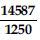will terminate after:

1. one decimal place
2. two decimal places
3. three decimal places
4. four decimal places

(d) four decimal places

##### Question 30:

Write whether every positive integer can be of the form 4q + 2, where q is an integer. Justify your answer.

No. For instance take 33 cannot be written in the form of 4q + 2 for any value of q as an integer.

##### Question 31:

‘‘The product of two consecutive positive integers is divisible by 2’’. Is this statement true or false? Give reasons.

‘‘The product of two consecutive positive integers is divisible by 2’’ is a true statement because one of them has to be even, and a multiple of an odd and an even integer is always even.
∵ Even integer is always divisible by 2.
∴ The product will also be divisible by 2.

##### Question 32:

‘‘The product of three consecutive positive integers is divisible by 6’’. Is this tatement true or false? Justify your answer.

As we know that three consecutive integers include an integer which is a multiple of 2 and an integer which is a multiple of 3.
∴ The product of three consecutive integers is divisible by 6. Hence, above statement is true.

##### Question 33:

Write whether the square of any positive integer can be of the form 3m + 2, where m is a natural number. Justify your answer.

The square of any positive integer cannot be written in the form 3m + 2, where m is a natural number.
As for an example,
Let the number is 4, its square is 16.
But 16 cannot be written as 3m + 2.
∵ 3m + 2 = 16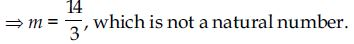##### Question 34:

A positive integer is of the form 3q + 1, q being a natural number. Can you write its square in any form other than 3m + 1, i.e., 3m or 3m + 2 for some integer m? Justify your answer.

No. Because for any positive integer of the form 3q + 1, its square will be (3q + 1)2 = 9q2 + 6q + 1 = 3(3q2 + 2q) + 1 = 3m + 1

##### Question 35:

The numbers 525 and 3000 are both divisible only by 3, 5, 15, 25 and 75. What is HCF (525, 3000)? Justify your answer.

∵ The numbers 525 and 3000 are both divisible only by 3, 5, 15, 25 and 75 only.
∴ HCF (525, 3000) = 75
∵ 75 is the highest amongst the common factors.

##### Question 36:

Explain why 3 x 5 x 7 + 7 is a composite number.

3 x 5 x 7 + 7 = 7(15 + 1)
= 7 x 16 = 7 x 2 x 8
Which has more than two factors.
∴ It is a composite number.

##### Question 37:

Can two numbers have 18 as their HCF and 380 as their LCM? Give reasons.

HCF must divide LCM but here 18 does not divide 380.
∴ Any two numbers cannot have 18 as their HCF and 380 as their LCM.

##### Question 38:

Without actually performing the long division, find if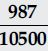will have terminating or non-terminating (repeating) decimal expansion. Give reasons for your answer.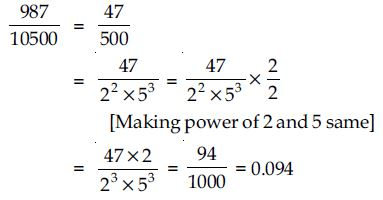##### Question 38:

A rational number in its decimal expansion is 327.7081. What can you say about the prime factors of q, when this number is expressed in the form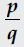Give reasons.

As 327.7081 is a terminating decimal number, so q must be of the form 2m5n, where m, n are natural numbers.

##### Question 39:

Show that the square of any positive integer is either of the form 4p or 4p + 1 for some integer p.

Any positive integer can be written as:
2n or 2n + 1 for some integer n.
Now, (2n)2 = 4n2
= 4p, where p = n2
and (2n + 1)2 = 4n2 + 4n + 1
= 4(n2 + n) + 1
= 4p + 1, p = n2 + n
So, square of any positive integer is either in the form 4p or 4p + 1.
So, square of any positive integer is either in the form 4p or 4p + 1.

##### Question 40:

Show that cube of any positive integer is of the form 4n, 4n + 1 or 4n + 3, for some integer n.

Any positive integer can be written as 4p, 4p + 1,
4p + 2, 4p + 3 for some integer p.
Now, (4p)3 = 64p3 = 4(16p3) = 4n,
where n = 16p3
(4p + 1)3 = 64p3 + 48p2 + 12p + 1
= 4(16p3 +12p2 + 3p) + 1 = 4n + 1,
where n = 16p3 + 12p2 + 3p
(4p + 2)3 = 64p3 + 96p2 + 48p + 8
= 4(16p3 + 24p2 + 12p + 2) = 4n,
where n = 16p3 + 24p2 + 12p + 2
(4p + 3)3 = 64p3 + 144p2 + 108p + 27
= 64p3 + 144p2 + 108p + 24 + 3
= 4(16p3 + 36p2 + 27p + 6) + 3
= 4n + 3,
where n = 16p3 + 36p2 + 27p + 6
So, cube of any positive integer is in the form 4n,
4n + 1 or 4n + 3, for some integer n.

##### Question 41:

Show that the square of any positive integer cannot be of the form 5p + 2 or 5p + 3 for any integer p.

Since square of any positive integer is of the form 4p or 4p + 1.
So, square of any positive integer cannot be in the form 5p + 2 or 5p + 3 for any integer p.

##### Question 42:

Show that the square of any positive integer cannot be of the form 6n + 2 or 6n + 5 for any integer n.

Any positive integer cannot be of the form 6n + 2 or 6n + 5 for any integer n.

##### Question 43:

Show that the square of any odd integer is of the form 4p + 1, for some integer p.

Any integer is of the form 2n + 1.
Now, (2n + 1)2 = 4n2 + 4n + 1
= 4(n2 + n) + 1
= 4p + 1, where p = n2 + n
So, square of any odd integer is in the form 4p + 1.

##### Question 44:

If n is an odd integer, then show that n2 - 1 is divisible by 8.

Any odd integer can be written in form of 2p + 1,
for some integer p.
Let n = 2p + 1
Now, n2 - 1 = (2p + 1)2 - 1
= 4p2 + 4p + 1 - 1
= 4p2 + 4p
= 4(p2 + p)
Since, p2 + p = p(p + 1)
So, if p is odd then p + 1 is even, and if p is even
then p + 1 is odd.
$⇒$In any case p2 + p is divisible by 2.
So, n2 - 1 is always divisible by 8.

##### Question 45:

Prove that if x and y are both odd positive integers, then x2 + y2 is even but not divisible by 4.

x and y are both odd positive integers.
∴ Let x = 2p + 1
and y = 2q + 1, for some integers p and q.
Now, x2 + y2 = (2p + 1)2 + (2q + 1)2
= 4p2 + 4p + 1 + 4q2 + 4q + 1
= 4p2 + 4q2 + 4p + 4q + 2
= 4(p2 + q2 + p + q) + 2
$⇒$ x2 + y2 is an even number but not divisible by 4.

##### Question 46:

Use Euclid’s division algorithm to find the HCF of 441, 567 and 693.

Here, 441 $<$ 567 $<$ 693.
Let us first take 441 and 567.
567 = 441 x 1 + 126
441 = 126 x 3 + 63
126 = 63 x 2 + 0
So, HCF of 567 and 441 = 63
Now, take 63 and 693.
693 = 63 x 11 + 0
So, HCF of 441, 567 and 693 = 63.

##### Question 47:

Using Euclid’s division algorithm to find the largest number that divides 1251, 9377 and 15628 leaving remainders 1, 2 and 3, respectively.

The largest number that divides 1251, 9377 and 15628 leaving remainders 1, 2 and 3 respectively is the HCF of (1251 - 1), (9377 - 2) and (15628 - 3).
So, we have to find HCF of 1250, 9375 and 15625.
9375 = 1250 x 7 + 625
1250 = 625 x 2 + 0
∴ HCF of 9375 and 1250 = 625.
Now HCF of 625 and 15625.
15625 = 625 x 25 + 0
So, HCF of 1250, 9375 and 15625 = 625
∴ The largest number that divides 1251, 9377 and 15628 leaving remainders 1, 2 and 3 respectively is 625.

##### Question 48: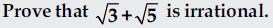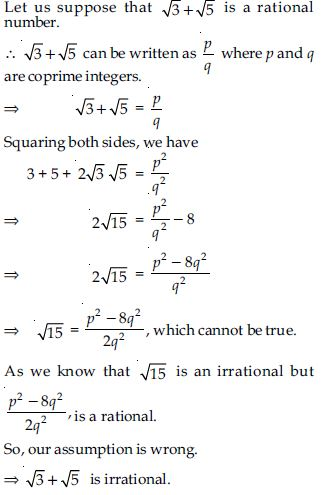##### Question 49:

Prove that 12n cannot end with the digit 0 or 5 for any natural number n.

12n can be written as (22 x 3)n = 22n x 3n. We know that any number can end with the digit 0 or 5 only if its factor are 5 and 2. ∴ 12n cannot end at 0 or 5.

##### Question 50:

On a morning walk, three persons step off together and their steps measure 40 cm, 42 cm and 45 cm, respectively. What is the minimum distance each should walk so that each can cover the same distance in complete steps?

Measures of steps of three persons are 40 cm, 42 cm and 45 cm, respectively.
If they have to cover same distance in complete steps.
We have to find LCM of their measures of steps.
LCM of 40, 42 and 45
Now, 40 = 23 x 5
42 = 2 x 3 x 7
45 = 32 x 5
∴ LCM of 40, 42 and 45
= 23 x 32 x 5 x 7 = 2520
∴ The distance they cover in complete steps
= 2520 cm = 25 m 20 cm.

##### Question 51:

Write the denominator of the rational number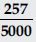in the form 2m x 5n, where m, n are nonnegative integers. Hence, write its decimal expansion, without actual division.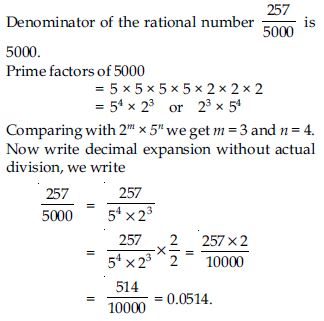##### Question 52: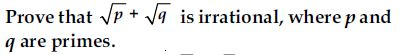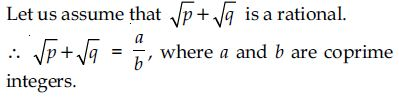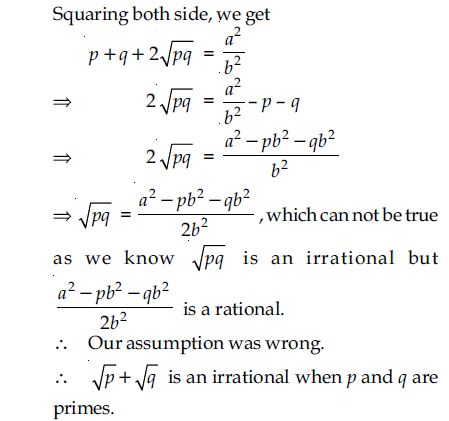##### Question 53:

Show that the cube of a positive integer of the form 6q + r, q is an integer and r = 0, 1, 2, 3, 4, 5 is also of the form 6m + r.

All positive integers can be written in the form of 6n + k, where n is any positive integer and k can be any integer 0, 1, 2, 3, 4, 5.
Now, the number can be written as 6n, 6n + 1,
6n + 2, 6n + 3, 6n + 4, 6n + 5.
∴ (6n)3 = 216n3 = 6(36n3)
= 6q + 0, where q = 36n3 and r = 0
(6n + 1)3 = 216n3 + 108n2 + 18n + 1
= 6(36n3 + 18n2 + 3n) + 1
= 6q + 1, where q = 36n3 + 18n2 + 3n and r = 1
(6n + 2)3 = 216n3 + 216n2 + 72n + 8
= 216n3 + 216n2 + 72n + 6 + 2
= 6(36n3 + 36n2 + 12n + 1) + 2
= 6q + 2,
where q = 36n3 + 36n2 + 12n + 1 and r = 2
(6n + 3)3 = 216n3 + 324n2 + 162n + 27
= 216n3 + 324n2 + 162n + 24 + 3
= 6(36n3 + 54n2 + 27n + 4) + 3
= 6q + 3,
where q = 36n3 + 54n2 + 27n + 4 and r = 3
(6n + 4)3 = 216n3 + 432n2 + 288n + 64
= 216n3 + 432n2 + 288n + 60 + 4
= 6(36n3 + 72n2 + 48n + 10) + 4
= 6q + 4,
where q = 36n3 + 72n2 + 48n + 10 and r = 4
(6n + 5)3 = 216n3 + 540n2 + 450n + 125
= 216n3 + 540n2 + 450n + 120 + 5
= 6(36n3 + 90n2 + 75n + 20) + 5
= 6q + 5,
where q = 36n3 + 90n2 + 75n + 20 and r = 5
So, by replacing q by m we can get the number to written as 6m + 0, 6m + 1, 6m + 2, 6m + 3, 6m + 4 and 6m + 5.

##### Question 54:

Prove that one and only one out of n, n + 2 and n + 4 is divisible by 3, where n is any positive integer.

Let ‘a’ be any positive integer and b = 3
Using Euclid's Lemma,
a = 3 x q + r, 0 $\le$ r $<$ 3
Case I: r = 0
a = 3q
(a) n = 3q (divisible by 3)
(b) n + 2 = 3q + 2 (not divisible by 3)
(c) n + 4 = 3q + 4 = 3q + 3 + 1
= 3(q + 1) + 1 (not divisible by 3)
Case II: r = 1
a = 3q + 1
(a) n = 3q + 1 (not divisible by 3)
(b) n + 2 = 3q + 3
= 3(q + 1) (divisible by 3)
(c) n + 4 = 3q + 5
= 3q + 3 + 2
= 3(q + 1) + 2 (not divisible by 3)
Case III: r = 2
a = 3q + 2
(a) n = 3q + 2 (not divisible by 3)
(b) n = 3q + 4
= 3q + 3 + 1
= 3(q + 1) + 1 (not divisible by 3)
(c) n = 3q + 6
= 3(q + 2), (divisible by 3)
So, it is clearly seen that only one out of n, n + 2,
n + 4 is divisible by 3 for a positive integer ‘n’.

##### Question 55:

Prove that one of any three consecutive positive integers must be divisible by 3.

Let the 3 consecutive integers are n, n + 1, n + 2.
Let ‘a’ be any positive integer and b = 3.
Using Euclid‘s Lemma,
a = b x q + r 0 ≤ r < b
Case I: r = 0
a = 3q
(a) n = 3q (divisible by 3)
(b) n + 1 = 3q + 1 (not divisible by 3)
(c) n + 2 = 3q + 2 (not divisible by 3)
Case II: r = 1
a = 3q + 1
(a) n = 3q + 1 (not divisible by 3)
(b) n + 1 = 3q + 2 (not divisible by 3)
(c) n + 2 = 3q + 3
= 3(q + 1) (divisible by 3)
Case III: r = 2
a = 3q + 2
(a) n = 3q + 2 (not divisible by 3)
(b) n + 1 = 3q + 3
= 3(q + 1) (divisible by 3)
(c) n + 2 = 3q + 4
= 3(q + 1) + 1 (not divisible by 3)

##### Question 56:

For any positive integer n, prove that n3 - n is divisible by 6.

Here, n3 - n = n(n2 - 1)
= n(n - 1) (n + 1),
which is three consecutive integers.
Let ‘a’ be any positive integer and b = 6
Using Euclid’s Lemma,
a = b x q + r, 0 $\le$ r $<$ b
Case I: r = 0
∴ a = 6q
n3 - n = n(n - 1) (n + 1)
= 6q(6q - 1) (6q + 1) (divisible by 6)
Case II: r = 1
∴ a = 6q + 1
n3 - n = n(n - 1) (n + 1)
= (6q + 1) (6q + 1 - 1) (6q + 1 + 1)
= (6q + 1) (6q) (6q + 2)
(divisible by 6)
Case III: r = 2
∴ a = 6q + 2
n3 - n = (n) (n - 1) (n + 1)
= (6q + 2) (6q + 2 - 1) (6q + 2 + 1)
= (6q + 2) (6q + 1) (6q + 3)
= 2(3q + 1) (6q + 1) 3(2q + 1)
= 6(3q + 1) (6q + 1) (2q + 1)
(divisible by 6)
Case IV: r = 3
n3 - n = n(n - 1) (n + 1)
= (6q + 3) (6q + 3 - 1) (6q + 3 + 1)
= 3(2q + 1) (6q + 2) (6q + 4)
= 6(2q + 1)(3q + 1)(6q + 4)
(divisible by 6)
Case V: r = 4
n3 - n = n(n - 1) (n + 1)
= (6q + 4) (6q + 4 - 1) (6q + 4 + 1)
= 2(3q + 2) (6q + 3) (6q + 5)
= 6(3q + 2) (2q + 1) (6q + 5)
(divisible by 6)
Case VI: r = 5
n3 - n = n(n - 1) (n + 1)
= (6q + 5) (6q + 5 - 1) (6q + 5 + 1)
= (6q + 5) (6q + 4) (6q + 6)
= 6(6q + 5) (6q + 4) (q + 1)
(divisible by 6)

##### Question 57:

Show that one and only one out of n, n + 4, n + 8, n + 12 and n + 16 is divisible by 5, where n is any positive integer.
[Hint: Any positive integer can be written in the form 5q, 5q + 1, 5q + 2, 5q + 3, 5q + 4].

Let a be any positive integer.
So, a can be written as 5q, 5q + 1, 5q + 2, 5q + 3,
5q + 4 for some integer q.
Suppose, n = 5q (divisible by 5)
n + 4 = 5q + 4 (not divisible by 5)
n + 8 = 5q + 8
= 5q + 5 + 3
= 5(q + 1) + 3 (not divisible by 5)
n + 12 = 5q + 12 = 5q + 10 + 2
= 5(q + 2) + 2 (not divisible by 5)
n + 16 = 5q + 16 = 5q + 15 + 1
= 5(q + 3) + 1 (not divisible by 5)
Suppose, n = 5q + 1
∴ n = 5q + 1 (not divisible by 5)
n + 4 = 5q + 1 + 4 = 5q + 5
= 5(q + 1) (divisible by 5)
n + 8 = 5q + 1 + 8 = 5q + 9
= 5q + 5 + 4
= 5(q + 1) + 4 (not divisible by 5)
n + 12 = 5q + 1 + 12 = 5q + 13
= 5q + 10 + 3
= 5(q + 2) + 3 (not divisible by 5)
n + 16 = 5q + 1 + 16 = 5q + 17
= 5q + 15 + 2
= 5(q + 3) + 2 (not divisible by 5) Suppose, n = 5q + 2 ∴ n = 5q + 2 (not divisible by 5)
n + 4 = 5q + 6
= 5(q + 1) + 1 (not divisible by 5)
n + 8 = 5q + 10
= 5(q + 2) (divisible by 5)
n + 12 = 5q + 14
= 5(q + 2) + 4 (not divisible by 5)
n + 16 = 5q + 18
= 5(q + 3) + 3 (not divisible by 5)
Suppose, n = 5q + 3
∴ n = 5q + 3 (not divisible by 5)
n + 4 = 5q + 7
= 5(q + 1) + 2 (not divisible by 5)
n + 8 = 5q + 11
= 5(q + 2) + 1 (not divisible by 5)
n + 12 = 5q + 15
= 5(q + 3) (divisible by 5)
n + 16 = 5q + 19
= 5(q + 3) + 4 (not divisible by 5)
Suppose, n = 5q + 4
∴ n = 5q + 4 (not divisible by 5)
n + 4 = 5q + 8
= 5(q + 1) + 3 (not divisible by 5)
n + 8 = 5q + 12
= 5(q + 2) + 2 (not divisible by 5)
n + 12 = 5q + 16
= 5(q + 3) + 1 (not divisible by 5)
n + 16 = 5q + 20
= 5(q + 4) (divisible by 5)
So, one and only one out of n, n + 4, n + 8, n + 12 and n + 16 is divisible by 5.